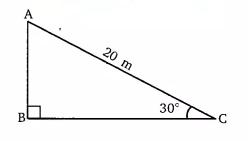Q

# A circus artist is climbing a 20 m long rope, which is tightly stretched and tied from the top of a vertical pole to the ground. Find the height of the pole, if the angle made by the rope with the ground level is 30° (see Fig. 9.11).

1. A circus artist is climbing a 20 m long rope, which is tightly stretched and tied from the top of a vertical pole to the ground. Find the height of the pole, if the angle made by the rope with the ground level is 30° (see Fig. 9.11).Views

Given that,
The length of the rope (AC) = 20 m. and
Let the height of the pole (AB) be

So, in the right triangleBy using the Sin rule

m.
Hence the height of the pole is 10 m.

Exams
Articles
Questions